Space, shape and measurement: Use and apply transformations to plot co-ordinates

# Unit 1: Transformation geometry

Dylan Busa### Unit outcomes: Unit 1: Transformation geometry

By the end of this unit you will be able to:

• Find the coordinates of the point $\scriptsize (x,y)$ after it is translated $\scriptsize p$ units horizontally and $\scriptsize q$ units vertically.
• Find the coordinates of the point $\scriptsize (x,y)$ after it is reflected about the x-axis, the y-axis, and the lines $\scriptsize y=x$and $\scriptsize y=-x$.

## What you should know

Before you start this unit, make sure you can:

## Introduction

In Unit 1 of Subject outcome 3.3 we learnt how to plot points and draw polygons on the Cartesian plane. The same process of plotting points on a plane very similar to the Cartesian plane is used by computer animators to create computer generated imagery (CGI) animated movies. Everything we see on screen is actually just thousands of carefully drawn polygons (mostly triangles).

But, these are movies. The polygons are not static. They move around the screen. Instead of drawing the polygons required for each frame, animators instead write rules for how a computer must change or transform each shape from frame to frame.

Although these are sophisticated transformations, they all fall into four broad categories:

• Translation – moving a point or a shape from one place to another.
• Reflection – creating a mirror image of a point or a shape about a given ‘mirror’ line or line of symmetry.
• Scaling – making a shape bigger (or smaller) without changing its shape.
• Rotation – turning a shape around a centre point by a given number of degrees.

In this unit, we are only going to explore translations and reflections. But before we do, spend some time exploring all four different types of transformations. To do this, you will need an internet connection. Visit the interactive simulation called Types of Transformations.Here you will be able to explore rotations, reflections, enlargements and translations. In each case, click and drag the blue points to alter the shape being transformed and see how the shape is being transformed.

### Note

To see each of these transformations in action, watch the video called “Rigid transformation intro”.

Rigid transformation intro (Duration: 07.22)## Translations

Translations are probably the simplest type of transformation we can do. We can translate (move) individual points or entire polygons or functions. When we translate a polygon or function, we are really just moving each point that makes up or defines the polygon or function.

Look at Figure 2. Here we have $\scriptsize \Delta \text{ABC}$ and the image of this triangle after translation which we call $\scriptsize \Delta \text{A }\!\!'\!\!\text{ B }\!\!'\!\!\text{ C }\!\!'\!\!\text{ }$ (A dash, B dash, C dash). We can see that the whole triangle has been translated vertically $\scriptsize 1$ unit up. We can also say that each of the vertices of the triangle has been translated vertically $\scriptsize 1$ unit up. The y-coordinate of each point has changed. We have added $\scriptsize 1$ to each y-coordinate.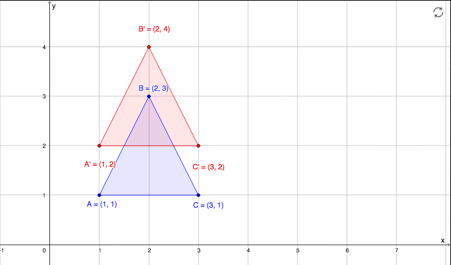Figure 2: Translation of $\scriptsize \Delta \text{ABC}$ $\scriptsize 1$ unit up

We can also translate $\scriptsize \Delta \text{ABC}$ horizontally, for example $\scriptsize 2$ units to the left, as show in Figure 3.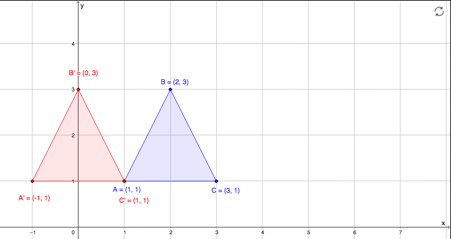Figure 3: Translation of $\scriptsize \Delta \text{ABC}$ $\scriptsize 2$ units to the left

In this case, we have subtracted $\scriptsize 2$ from the x-coordinate of each vertex.

Naturally, we can translate a point, shape or function both vertically and horizontally at the same time. How has $\scriptsize \Delta \text{ABC}$ been translated in Figure 4?Figure 4: Translation of $\scriptsize \Delta \text{ABC}$ $\scriptsize 2$ units to the right and $\scriptsize 1.5$ units down

If you have an internet connection, visit the interactive simulation called Translations to practise translating a triangle around the Cartesian plane.You can click and drag each vertex of the blue triangle to change its shape.### Example 1.1

What are the coordinates of $\scriptsize \text{A }\!\!'\!\!\text{ }$ if $\scriptsize \text{A}(3,-1)$ is translated $\scriptsize 3$ units down and $\scriptsize 6$ units to the right?

Solution

If $\scriptsize \text{A}(3,-1)$ is translated $\scriptsize 3$ units down, we need to subtract $\scriptsize 3$ from the y-coordinate of $\scriptsize \text{A}$. This coordinate will become $\scriptsize -1-3=-4$.

If $\scriptsize \text{A}(3,-1)$ is translated $\scriptsize 6$ units to the right, we need to add $\scriptsize 6$ to the x-coordinate of $\scriptsize \text{A}$. This coordinate will become $\scriptsize 3+6=9$.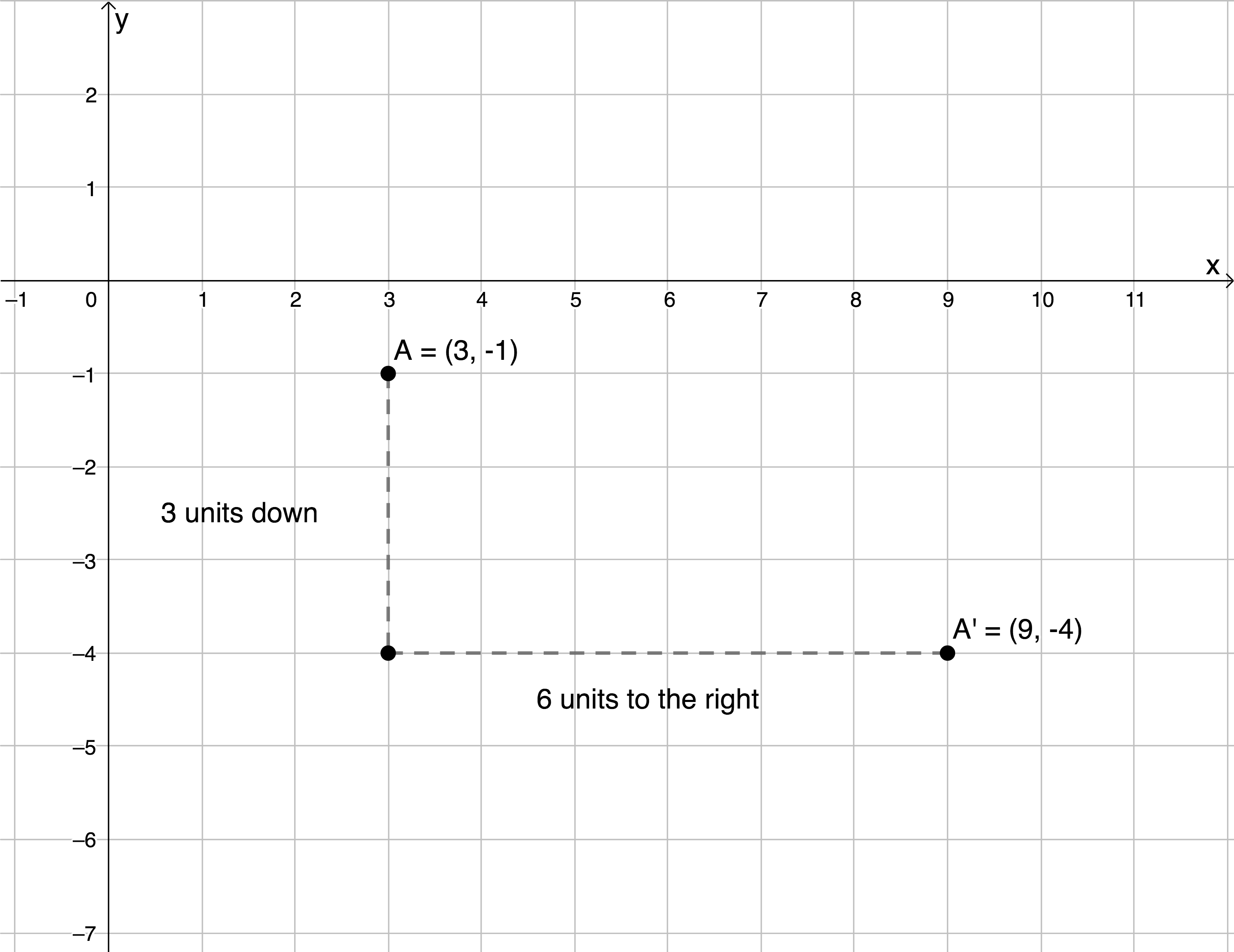Therefore $\scriptsize \text{A }\!\!'\!\!\text{ }$ will have coordinates $\scriptsize (9,-4)$.

### Note

Any vertical (up or down) movement of a point is reflected as a change in the point’s y-coordinate. As a point is moved up, its y-coordinate gets bigger.

Any horizontal (left or right ) movement of a point is reflected as a change in the point’s x-coordinate. As a point moves to the right, its x-coordinate gets bigger.### Exercise 1.1

1. Write down the coordinates of $\scriptsize \text{Q }\!\!'\!\!\text{ }$ if $\scriptsize \text{Q}(-1.5,5)$ is translated:
1. $\scriptsize 2$ units to the left.
2. $\scriptsize 8$ units up and $\scriptsize 5$ units to the right.
3. $\scriptsize 3$ units to the right and $\scriptsize 3$ units down.
4. $\scriptsize p$ units to the left and $\scriptsize q$ units up.
2. In the following diagram $\scriptsize \Delta \text{XYZ}$ has vertices as indicated. On the same set of axes, sketch $\scriptsize \Delta \text{XYZ}$ and $\scriptsize \Delta \text{X }\!\!'\!\!\text{ Y }\!\!'\!\!\text{ Z }\!\!'\!\!\text{ }$ if $\scriptsize \Delta \text{XYZ}$ is translated $\scriptsize 2$ units to the right and $\scriptsize 6$ units down.3. If $\scriptsize \text{G}'(-6,3)$, what are the coordinates of $\scriptsize \text{G}$ if $\scriptsize \text{G}$ was translated $\scriptsize 3$ units down and $\scriptsize 2$ units to the right.

The full solutions are at the end of the unit.

• To translate a point $\scriptsize p$ units to the right, add $\scriptsize p$ to the x-coordinate.
• To translate a point $\scriptsize p$ units to the left, subtract $\scriptsize p$ from the x-coordinate.
• To translate a point $\scriptsize q$ units up, add $\scriptsize q$ to the y-coordinate.
• To translate a point $\scriptsize p$ units down, subtract $\scriptsize q$ from the y-coordinate.

## Reflections

We all know what a reflection is. We see one every time we look in a mirror. We can use the same principle to reflect points, shapes and functions across different lines (or mirrors) on the Cartesian plane.

In this unit we will only look at reflections made across the following lines:

• The y-axis (the line $\scriptsize x=0$)
• The x-axis (the line $\scriptsize y=0$)
• The line $\scriptsize y=x$
• The line $\scriptsize y=-x$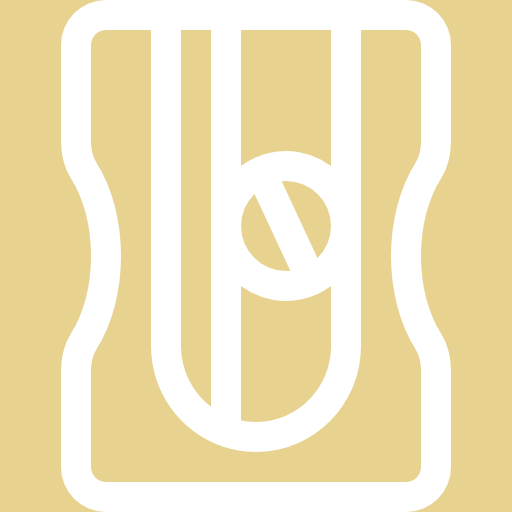### Activity 1.1: Reflect across the axes

Time required: 10 minutes

What you need:

• a piece of paper
• a pen or pencil

What to do:

1. The figure below shows $\scriptsize \text{CDEFGH}$ reflected across the y-axis to form the image $\scriptsize \text{C }\!\!'\!\!\text{ D }\!\!'\!\!\text{ E }\!\!'\!\!\text{ F }\!\!'\!\!\text{ G }\!\!'\!\!\text{ H }\!\!'\!\!\text{ }$. Write down the transformation of each vertex from $\scriptsize \text{CDEFGH}$ to $\scriptsize \text{C }\!\!'\!\!\text{ D }\!\!'\!\!\text{ E }\!\!'\!\!\text{ F }\!\!'\!\!\text{ G }\!\!'\!\!\text{ H }\!\!'\!\!\text{ }$.2. Describe the ‘rule’ that we can use to transform the coordinates of a point when reflected about the y-axis or the line $\scriptsize x=0$.
3. The following figure at shows $\scriptsize \text{CDEFGH}$ reflected across the x-axis to form the image $\scriptsize \text{C}{{\text{ }\!\!'\!\!\text{ }}_{1}}\text{D}{{\text{ }\!\!'\!\!\text{ }}_{1}}\text{E}{{\text{ }\!\!'\!\!\text{ }}_{1}}\text{F}{{\text{ }\!\!'\!\!\text{ }}_{1}}\text{G}{{\text{ }\!\!'\!\!\text{ }}_{1}}\text{H}{{\text{ }\!\!'\!\!\text{ }}_{1}}$. Write down the transformation of each vertex from $\scriptsize \text{CDEFGH}$ to $\scriptsize \text{C}{{\text{ }\!\!'\!\!\text{ }}_{1}}\text{D}{{\text{ }\!\!'\!\!\text{ }}_{1}}\text{E}{{\text{ }\!\!'\!\!\text{ }}_{1}}\text{F}{{\text{ }\!\!'\!\!\text{ }}_{1}}\text{G}{{\text{ }\!\!'\!\!\text{ }}_{1}}\text{H}{{\text{ }\!\!'\!\!\text{ }}_{1}}$.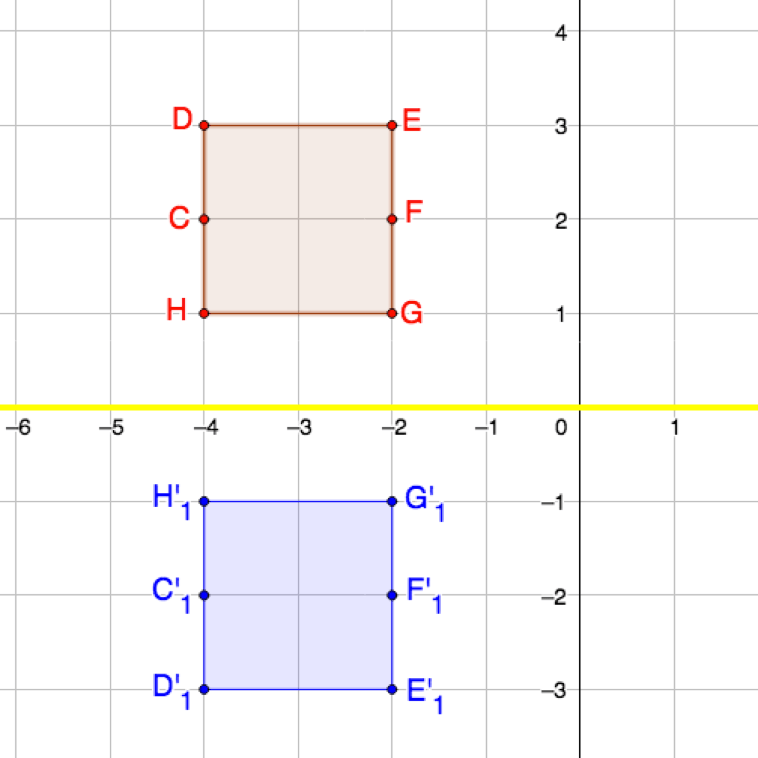4. Describe the ‘rule’ that we can use to transform the coordinates of a point when reflected about the x-axis or the line $\scriptsize y=0$.
5. If you have an internet connection, visit the interactive simulation called Reflections.Click and drag any/all of the vertices of $\scriptsize \text{CDEFGH}$ to change the shape of the polygon. Do your rules for transforming these points when the shape is reflected about the y-axis and the x-axis hold?

What did you find:

1. This is how each of the vertices was transformed:
$\scriptsize \text{C}(-3,2)\to \text{C}'(3,2)$
$\scriptsize \text{D}(-3,3)\to \text{D}'(3,3)$
$\scriptsize \text{E}(-1,3)\to \text{E}'(1,3)$
$\scriptsize \text{F}(-1,2)\to \text{F}'(1,2)$
$\scriptsize \text{G}(-1,1)\to \text{G}'(1,1)$
$\scriptsize \text{H}(-3,1)\to \text{H}'(3,1)$
2. In each case, the x-coordinate was multiplied by $\scriptsize -1$ while the y-coordinate remained the same.
3. This is how each of the vertices was transformed:
$\scriptsize \text{C}(-3,2)\to \text{C}{{'}_{1}}(-3,-2)$
$\scriptsize \text{D}(-3,3)\to \text{D}{{'}_{1}}(-3,-3)$
$\scriptsize \text{E}(-1,3)\to \text{E}{{'}_{1}}(-1,-3)$
$\scriptsize \text{F}(-1,2)\to \text{F}{{'}_{1}}(-1,-2)$
$\scriptsize \text{G}(-1,1)\to \text{G}{{'}_{1}}(-1,-1)$
$\scriptsize \text{H}(-3,1)\to \text{H}{{'}_{1}}(-3,-1)$
4. In each case, the y-coordinate was multiplied by $\scriptsize -1$ while the x-coordinate remained the same.
5. Here is a screenshot of the interactive simulation where the coordinates of all the vertices of $\scriptsize \text{CDEFGH}$ have been changed and the shape reflected across the y-axis..
We can see that the rule we discovered of simply multiplying the x-coordinate of each point by $\scriptsize -1$ still holds.
.
Here is another screenshot of the same simulation where the vertices of $\scriptsize \text{CDEFGH}$ have been changed again and the shape reflected about the x-axis. Once again, we can see that our rule of simply multiplying the y-coordinates of each point by $\scriptsize -1$ also still holds.We discovered in Activity 1.1 that when we reflect a point across the y-axis (the line $\scriptsize x=0$), all we need to do is change the sign of the x-coordinate (multiply it by $\scriptsize -1$) and leave the y-coordinate the same.

When we reflect a point across the x-axis (the line $\scriptsize y=0$), all we need to do is change the sign of the y-coordinate (multiply it by $\scriptsize -1$) and leave the x-coordinate the same.

When reflecting a point across the y-axis / the line $\scriptsize x=0$: $\scriptsize \text{A}(x,y)\to \text{A}'(-x,y)$

When reflecting a point across the x-axis / the line $\scriptsize y=0$: $\scriptsize \text{A}(x,y)\to \text{A}'(x,-y)$### Activity 1.2: Reflect across the line y= x

Time required: 10 minutes

What you need:

• a piece of paper
• a pen or pencil

What to do:

1. Below is $\scriptsize \text{CDEFGH}$ reflected across the line $\scriptsize y=x$ to form the image$\scriptsize \text{C}{{\text{ }\!\!'\!\!\text{ }}_{2}}\text{D}{{\text{ }\!\!'\!\!\text{ }}_{2}}\text{E}{{\text{ }\!\!'\!\!\text{ }}_{2}}\text{F}{{\text{ }\!\!'\!\!\text{ }}_{2}}\text{G}{{\text{ }\!\!'\!\!\text{ }}_{2}}\text{H}{{\text{ }\!\!'\!\!\text{ }}_{2}}$. Write down the transformation of each vertex from $\scriptsize \text{CDEFGH}$ to $\scriptsize \text{C}{{\text{ }\!\!'\!\!\text{ }}_{2}}\text{D}{{\text{ }\!\!'\!\!\text{ }}_{2}}\text{E}{{\text{ }\!\!'\!\!\text{ }}_{2}}\text{F}{{\text{ }\!\!'\!\!\text{ }}_{2}}\text{G}{{\text{ }\!\!'\!\!\text{ }}_{2}}\text{H}{{\text{ }\!\!'\!\!\text{ }}_{2}}$.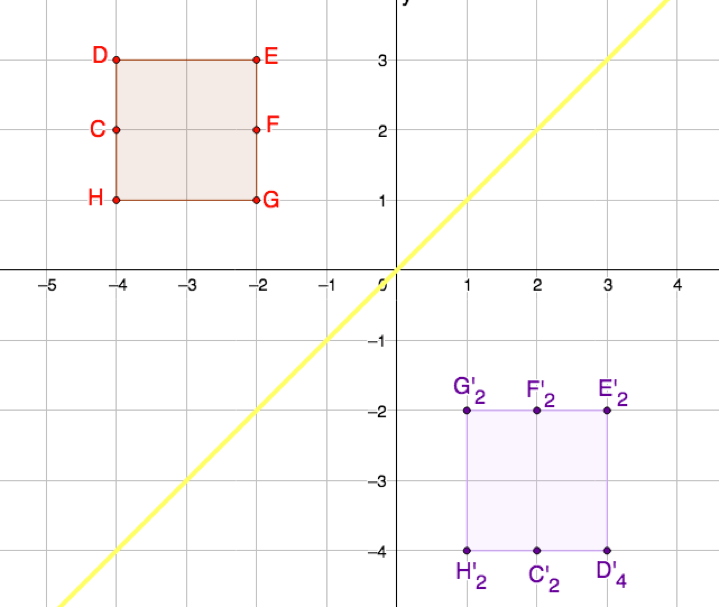2. Describe the ‘rule’ that we can use to transform the coordinates of a point when reflected about the line $\scriptsize y=x$.
3. What do you think the ‘rule’ would be when reflecting a point about the line $\scriptsize y=-x$?

What did you find:

1. This is how each of the vertices was transformed:
$\scriptsize \text{C}(-3,2)\to \text{C}{{'}_{2}}(2,-3)$
$\scriptsize \text{D}(-3,3)\to \text{D}{{'}_{2}}(3,-3)$
$\scriptsize \text{E}(-1,3)\to \text{E}{{'}_{2}}(3,-1)$
$\scriptsize \text{F}(-1,2)\to \text{F}{{'}_{2}}(2,-1)$
$\scriptsize \text{G}(-1,1)\to \text{G}{{'}_{2}}(1,-1)$
$\scriptsize \text{H}(-3,1)\to \text{H}{{'}_{2}}(1,-3)$
2. In each case, the x- and y-coordinates were swopped around. In other words, the point $\scriptsize (x,y)$ was transformed into the point $\scriptsize (y,x)$. This makes sense given that we are reflecting across the line $\scriptsize y=x$. The new y-coordinate is equal to the old x-coordinate. The new x-coordinate is equal to the old y-coordinate.
3. If we think about reflecting a point across the line $\scriptsize y=-x$ we will need to swop the coordinates around and change their signs (in other words multiply them by $\scriptsize -1$). So, the point $\scriptsize (x,y)$ will become the point $\scriptsize (-y,-x)$. The figure below shows what the image of $\scriptsize \text{CDEFGH}$ looks like when reflected across the line $\scriptsize y=-x$.We discovered in Activity 1.2 that when we reflect a point across the line $\scriptsize y=x$, we need to swop the coordinates around to find the reflected point.

When we reflect a point across the line $\scriptsize y=-x$, we need to swop the coordinates and multiply them by $\scriptsize -1$ to find the reflected point.

If you have an internet connection, spend some time playing with the interactive simulation called Reflections.Click and drag any/all of the vertices of $\scriptsize \text{CDEFGH}$ to change the shape of the polygon and see how these points are transformed when reflected across the lines $\scriptsize y=x$ and $\scriptsize y=-x$.

When reflecting a point across the line $\scriptsize y=x$: $\scriptsize \text{A}(x,y)\to \text{A}'(y,x)$

When reflecting a point across the line $\scriptsize y=-x$: $\scriptsize \text{A}(x,y)\to \text{A}'(-y,-x)$### Example 1.2

What are the coordinates of $\scriptsize \text{A }\!\!'\!\!\text{ }$ if $\scriptsize \text{A}(3,-1)$ is reflected across:

1. the y-axis
2. the x-axis
3. the line $\scriptsize y=x$
4. the line $\scriptsize y=-x$

Solutions

1. When reflecting a point across the y-axis, we transform the point as follows: $\scriptsize \text{A}(x,y)\to \text{A}'(-x,y)$. Therefore, $\scriptsize \text{A}(3,-1)\to \text{A}'(-3,-1)$.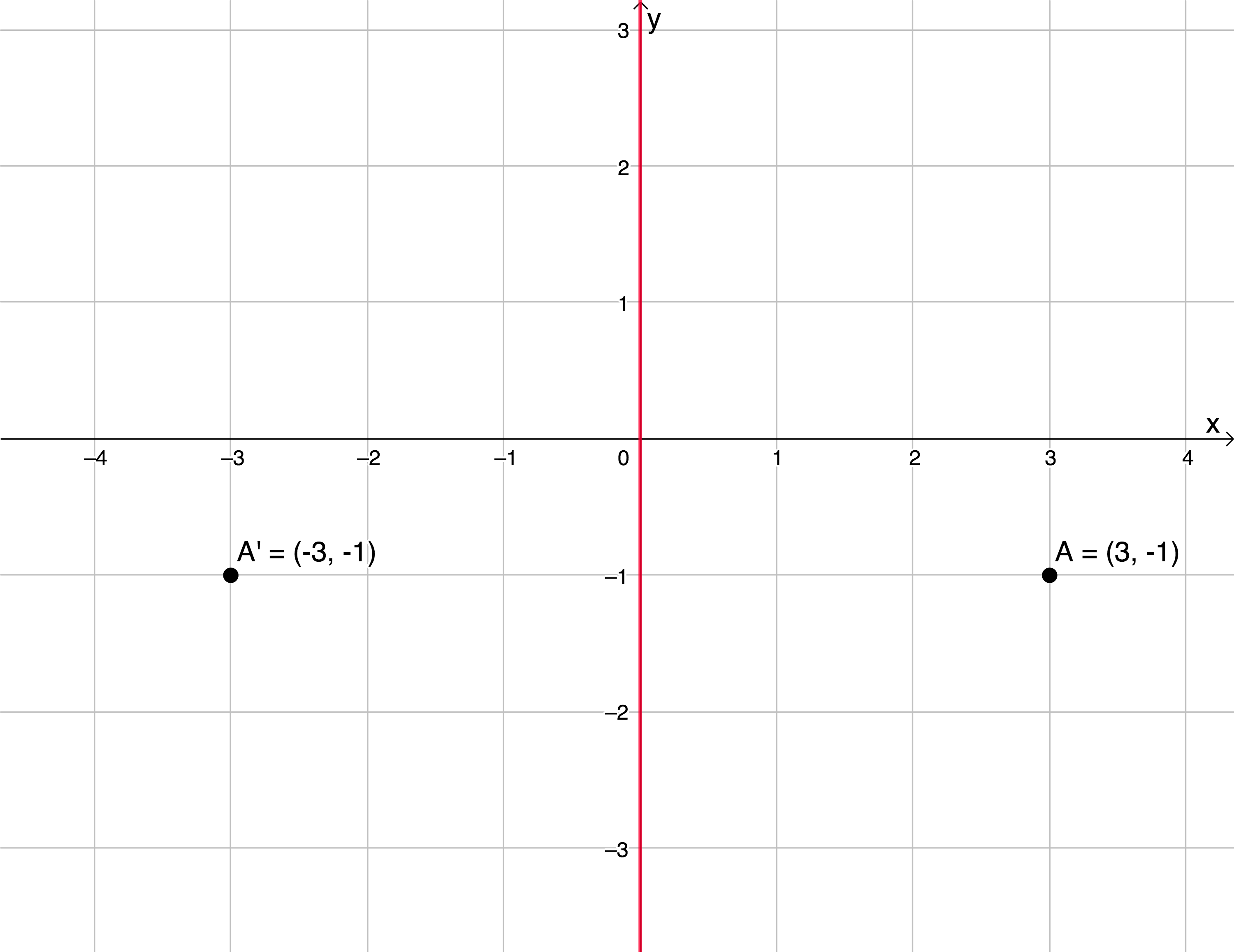2. When reflecting a point across the x-axis, we transform the point as follows: $\scriptsize \text{A}(x,y)\to \text{A}'(x,-y)$. Therefore, $\scriptsize \text{A}(3,-1)\to \text{A}'(3,1)$.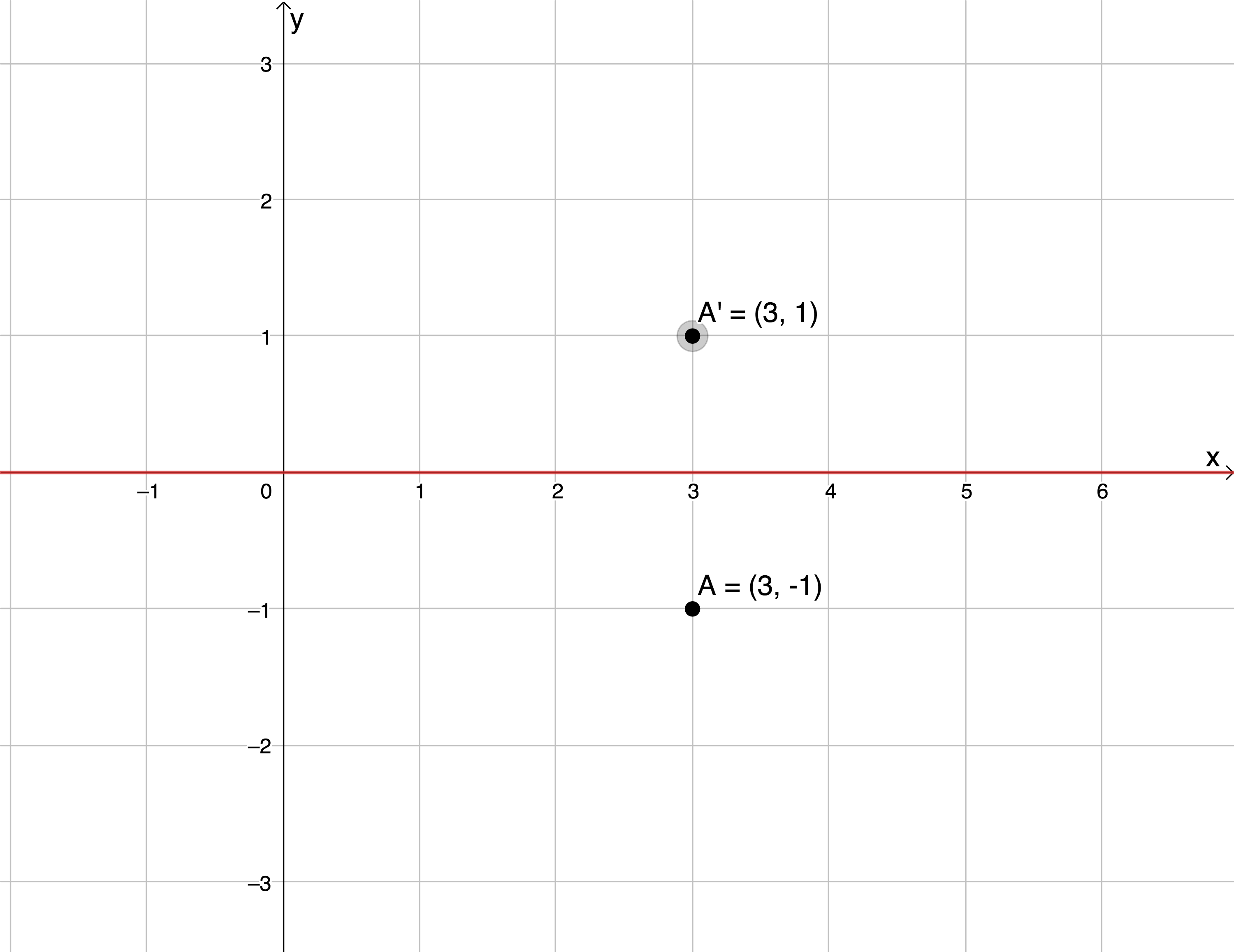3. When reflecting a point across the line $\scriptsize y=x$, we transform the point as follows: $\scriptsize \text{A}(x,y)\to \text{A}'(y,x)$. Therefore, $\scriptsize \text{A}(3,-1)\to \text{A}'(-1,3)$.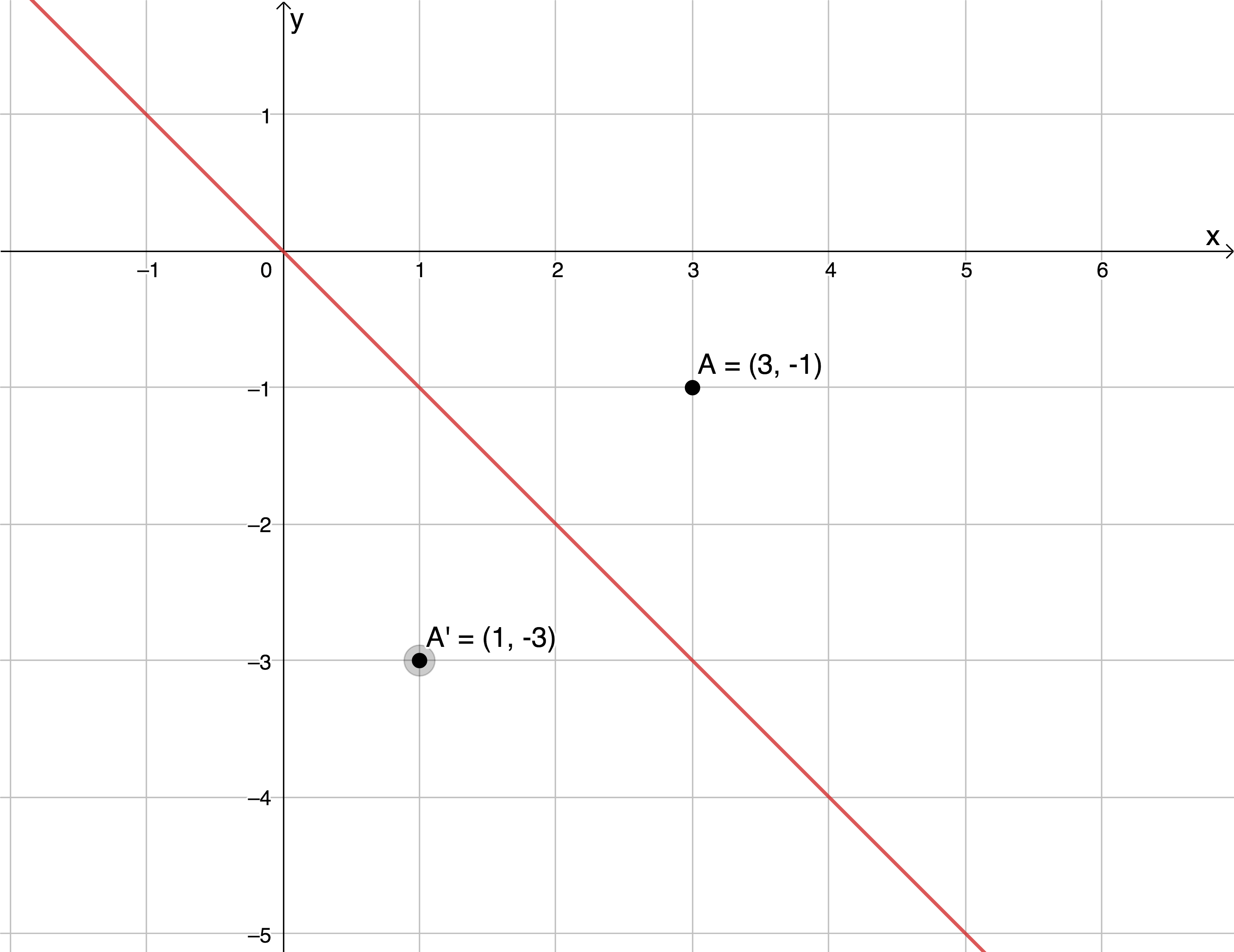4. When reflecting a point across the line $\scriptsize y=-x$, we transform the point as follows: $\scriptsize \text{A}(x,y)\to \text{A}'(-y,-x)$. Therefore, $\scriptsize \text{A}(3,-1)\to \text{A}'(1,-3)$.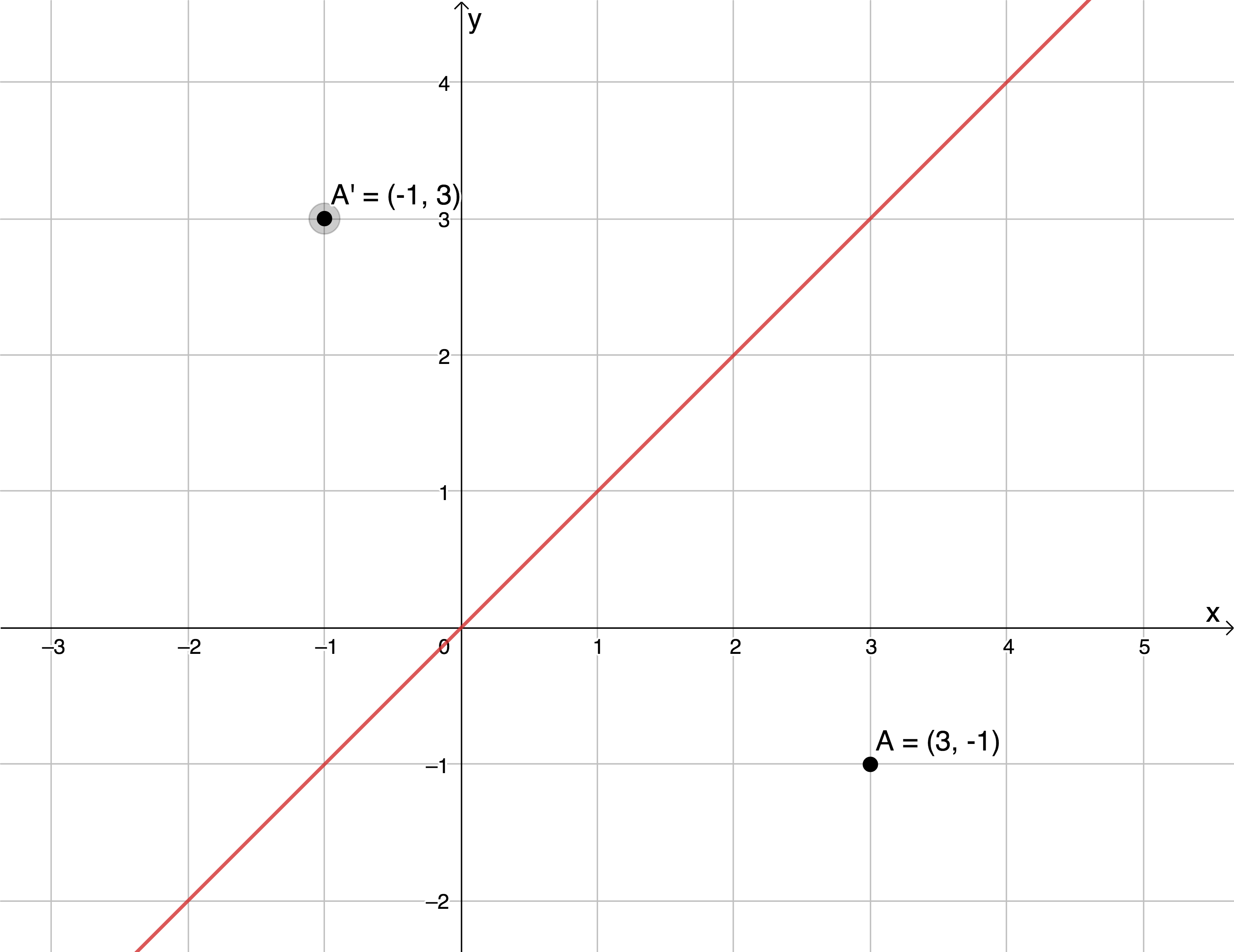### Exercise 1.2

1. $\scriptsize \Delta \text{ABC}$ on the Cartesian plane is shown in the diagram below. On the same set of axes, sketch $\scriptsize \Delta \text{ABC}$ and the image $\scriptsize \Delta \text{A }\!\!'\!\!\text{ B }\!\!'\!\!\text{ C }\!\!'\!\!\text{ }$ if $\scriptsize \Delta \text{ABC}$ is reflected across:
1. the x-axis.
2. the line $\scriptsize y=-x$.2. If $\scriptsize \text{D }\!\!'\!\!\text{ }(-6,-2)$ is a reflection of $\scriptsize \text{D}$ across the line $\scriptsize y=x$, what are the coordinates of $\scriptsize \text{D}$?

The full solutions are at the end of the unit.

## Summary

In this unit you have learnt the following:

• How to find the coordinates of the point $\scriptsize (x,y)$ after it is translated $\scriptsize p$ units horizontally and $\scriptsize q$ units vertically.
• How to find the coordinates of the point $\scriptsize (x,y)$ after it is reflected about the x-axis, the y-axis, and the lines $\scriptsize y=x$and $\scriptsize y=-x$.

# Unit 1: Assessment

#### Suggested time to complete: 10 minutes

1. The following diagram shows an image of a cartoon character. Three points A, B and C are found on the diagram. Use the sketch given below and answer the questions that follow.1. What would be the co-ordinates of point A if it were translated $\scriptsize 3$ units up and $\scriptsize 2$ units to the right?
2. What would be the co-ordinates of point B if it were reflected across the line $\scriptsize y=-x$?
3. What would be the co-ordinates of point A if it is reflected across the y-axis?
4. What would be the co-ordinates of point C if it is reflected across the line $\scriptsize y=x$?
• $\scriptsize \text{A}(x,y)$ is a point on the Cartesian plane. Without sketching, write down the coordinates of the image of $\scriptsize \text{A}$ ($\scriptsize \text{A}'$) if $\scriptsize \text{A}$ is shifted $\scriptsize 1$ unit to the left, then $\scriptsize 3$ units up then reflected across the line $\scriptsize y=x$.

The full solutions are at the end of the unit.

# Unit 1: Solutions

### Exercise 1.1

1. .
1. If $\scriptsize \text{Q}(-1.5,5)$ is translated $\scriptsize 2$ units to the left, then we need to subtract $\scriptsize 2$ units from the x-coordinate. There is no vertical translation, so the y-coordinate does not change. $\scriptsize \text{Q}(-1.5,5)\to \text{Q }\!\!'\!\!\text{ }(-3.5,5)$
2. If $\scriptsize \text{Q}(-1.5,5)$ is translated $\scriptsize 8$ units up and $\scriptsize 5$ units to the right, then we need to add $\scriptsize 8$ units to the y-coordinate and add $\scriptsize 5$ units to the x-coordinate. $\scriptsize \text{Q}(-1.5,5)\to \text{Q }\!\!'\!\!\text{ }(3.5,13)$
3. If $\scriptsize \text{Q}(-1.5,5)$ is translated $\scriptsize 3$ units to the right and $\scriptsize 3$ units down, then we need to add $\scriptsize 5$ units to the x-coordinate and subtract $\scriptsize 3$ units from the y-coordinate. $\scriptsize \text{Q}(-1.5,5)\to \text{Q }\!\!'\!\!\text{ }(1.5,2)$
4. If $\scriptsize \text{Q}(-1.5,5)$ is translated $\scriptsize p$ units to the left and $\scriptsize q$ units up, then we need to subtract $\scriptsize p$ units from the x-coordinate and add $\scriptsize q$ units to the y-coordinate. $\scriptsize \text{Q}(-1.5,5)\to \text{Q }\!\!'\!\!\text{ }(-1.5-p,5+q)$
2. $\scriptsize \Delta \text{XYZ}$ is translated $\scriptsize 2$ units to the right and $\scriptsize 6$ units down. Therefore, each vertex is translated $\scriptsize 2$ units to the right and $\scriptsize 6$ units down.
$\scriptsize \text{X}(-2,3)\to \text{X }\!\!'\!\!\text{ }(0,-3)$
$\scriptsize \text{Y}(-1,-2)\to \text{Y }\!\!'\!\!\text{ }(1,-8)$
$\scriptsize \text{Z}(4,-1)\to \text{Z }\!\!'\!\!\text{ }(6,-7)$3. Because we are starting with $\scriptsize \text{G}'(-6,3)$ and need to get back to $\scriptsize \text{G}$ we have to perform the normal translations in reverse. $\scriptsize \text{G}$ was translated $\scriptsize 3$ units down and $\scriptsize 2$ units to the right. Therefore, we need to add $\scriptsize 3$ units to the y-coordinate of $\scriptsize \text{G }\!\!'\!\!\text{ }$ and subtract $\scriptsize 2$ units from the x-coordinate of $\scriptsize \text{G }\!\!'\!\!\text{ }$.
$\scriptsize \text{G}'(-6,3)\to G(-8,6)$

Back to Exercise 1.1

### Exercise 1.2

1. .
1. $\scriptsize \Delta \text{ABC}$ is reflected across the x-axis. Therefore, the vertices are transformed as follows:
$\scriptsize \text{A}(-1,1)\to \text{A}'(-1,-1)$
$\scriptsize \text{B}(2,1)\to \text{B}'(2,-1)$
$\scriptsize \text{C}(1,-1)\to \text{C}'(1,1)$2. $\scriptsize \Delta \text{ABC}$ is reflected across the line $\scriptsize y=-x$ . Therefore, the vertices are transformed as follows:
$\scriptsize \text{A}(-1,1)\to \text{A}'(-1,1)$
$\scriptsize \text{B}(2,1)\to \text{B}'(-1,-2)$
$\scriptsize \text{C}(1,-1)\to \text{C}'(1,-1)$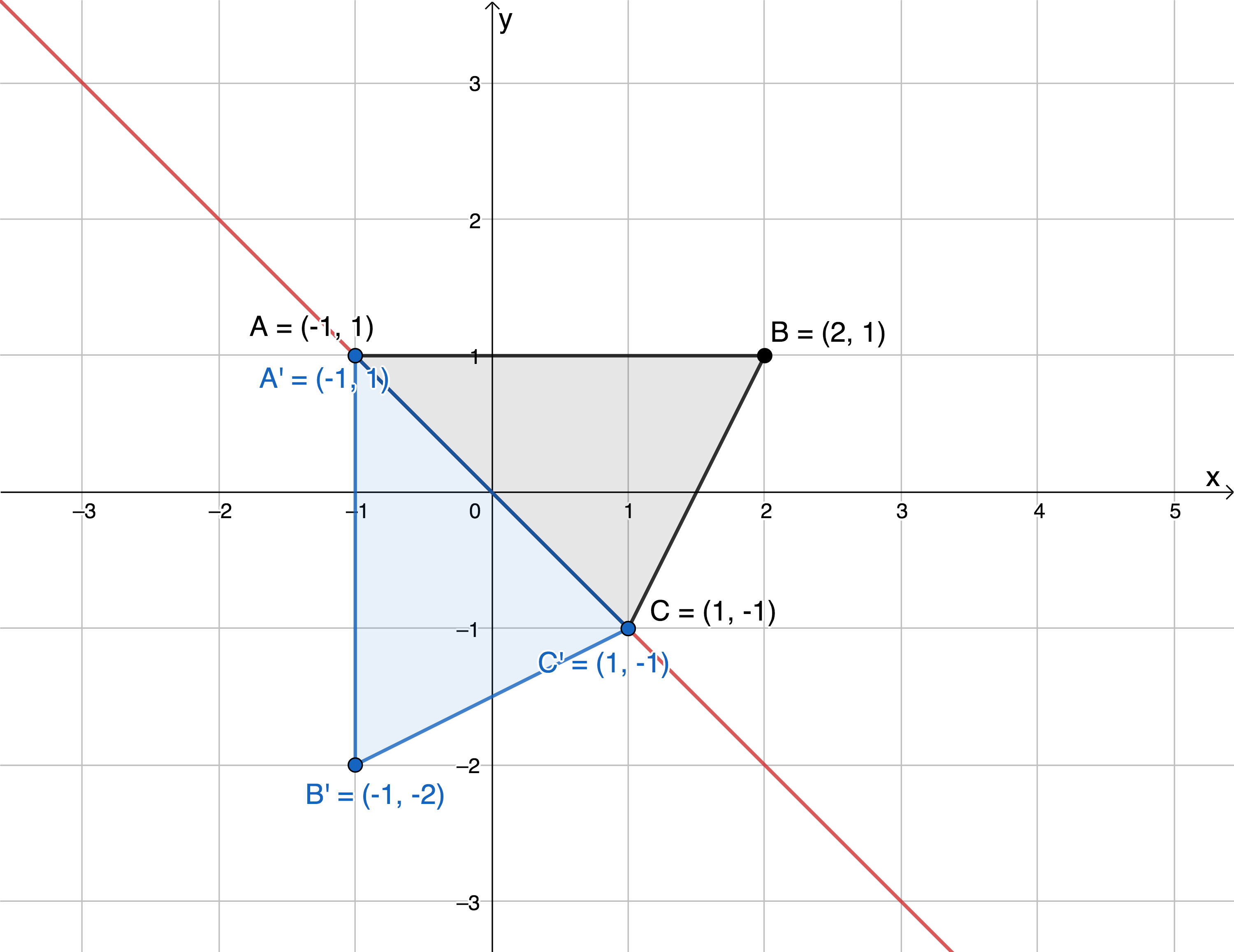2. $\scriptsize \text{D }\!\!'\!\!\text{ }(-6,-2)$ is a reflection of $\scriptsize \text{D}$ across the line $\scriptsize y=x$. We know that $\scriptsize \text{D}(x,y)\to \text{D}'(y,x)$. Therefore $\scriptsize \text{D}(-2,-6)$.

Back to Exercise 1.2

### Unit 1: Assessment

1. .
1. $\scriptsize \text{A}(8,-2)$ is translated $\scriptsize 3$ units up and $\scriptsize 2$ units to the right. $\scriptsize \text{A}(x,y)\to \text{A}'(x+p,y+q)$. Therefore, $\scriptsize \text{A}'(10,1)$.
2. $\scriptsize \text{B}(6,-2)$ reflected across the line $\scriptsize y=-x$. $\scriptsize \text{B}(x,y)\to \text{B }\!\!'\!\!\text{ }(-y,-x)$. Therefore $\scriptsize \text{B }\!\!'\!\!\text{ }(2,-6)$.
3. $\scriptsize \text{A}(8,-2)$ is reflected across the y-axis. $\scriptsize \text{A}(x,y)\to \text{A }\!\!'\!\!\text{ }(-x,y)$. Therefore $\scriptsize \text{A }\!\!'\!\!\text{ }(-8,-2)$.
4. $\scriptsize \text{C}(3,-7)$ reflected across the line $\scriptsize y=x$. $\scriptsize \text{C}(x,y)\to \text{C }\!\!'\!\!\text{ }(y,x)$. Therefore $\scriptsize \text{C }\!\!'\!\!\text{ }(-7,3)$.
2. $\scriptsize \text{A}(x,y)$
Translation $\scriptsize 1$ unit to the left: $\scriptsize \text{A}(x,y)\to \text{A}'(x-1,y)$
Translation $\scriptsize 3$ units up: $\scriptsize \text{A }\!\!'\!\!\text{ }(x-1,y)\to \text{A}'(x-1,y+3)$
Reflection across the line $\scriptsize y=x$: $\scriptsize \text{A}'(x-1,y+3)\to \text{A}'(y+3,x-1)$

Back to Unit 1: Assessment